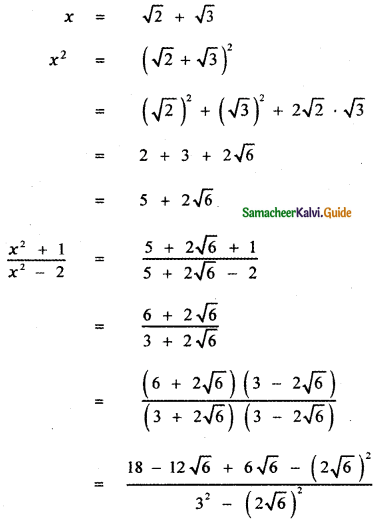Tamilnadu State Board New Syllabus Samacheer Kalvi 11th Maths Guide Pdf Chapter 2 Basic Algebra Ex 2.11 Text Book Back Questions and Answers, Notes.

## Tamilnadu Samacheer Kalvi 11th Maths Solutions Chapter 2 Basic Algebra Ex 2.11

Question 1.
Simplify
(a) (125)2/3
(b) 16-3/4
(c) (- 1000)-2/3
(d) (3-6)1/3
(e) $$\frac{27^{-\frac{2}{3}}}{27^{-\frac{1}{3}}}$$
(a) (125)2/3(b) 16-3/4(c) (- 1000)-2/3(d) (3-6)1/3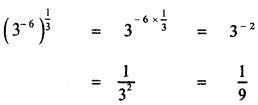(e) $$\frac{27^{-\frac{2}{3}}}{27^{-\frac{1}{3}}}$$Question 2.
Evaluate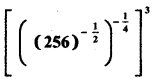Question 3.
If $$\left(x^{\frac{1}{2}}+x^{-\frac{1}{2}}\right)^{2}=\frac{9}{2}$$ then find the value of $$\left(x^{\frac{1}{2}}-x^{-\frac{1}{2}}\right)$$ for x > 1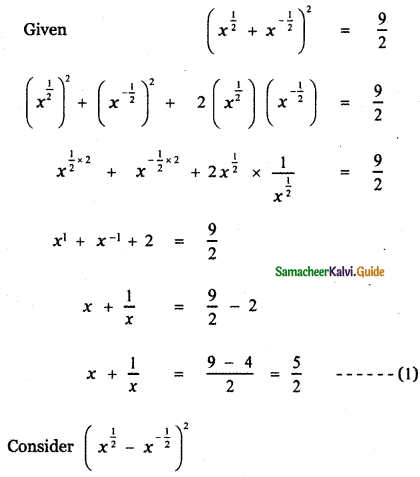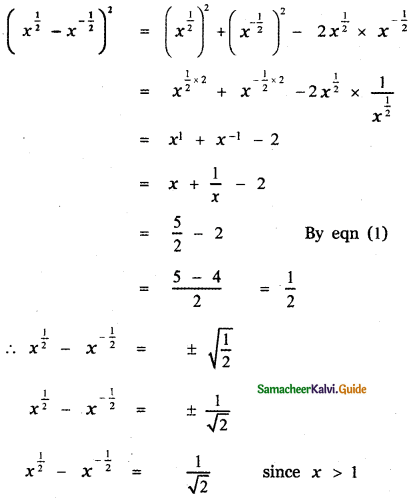Question 4.
Simplify and hence find the value of n:
$$\frac{3^{2 n} \cdot 9^{2} \cdot 3^{-n}}{3^{3 n}}$$ = 27
Given4 – 2n = 3
2n = 4 – 3
2n = 1
n = $$\frac { 1 }{ 2 }$$

Question 5.
Find the radius of the spherical tank whose volume is $$\frac{32 \pi}{3}$$ units.
Let r be the radius of the spherical tank.
Given volume of the spherical tank = $$\frac{32 \pi}{3}$$
$$\frac{4}{3}$$πr3 = $$\frac{32 \pi}{3}$$
4r3 = 32
r3 = $$\frac{32}{4}$$ = 8
r3 = 23
r = 2
∴ Radius of the spherical tank r = 2 units.Question 6.
Simplify by rationalizing the denominator $$\frac{7+\sqrt{6}}{3-\sqrt{2}}$$
$$\frac{7+\sqrt{6}}{3-\sqrt{2}}$$
Multiply the numerator and denominator by 3 + √2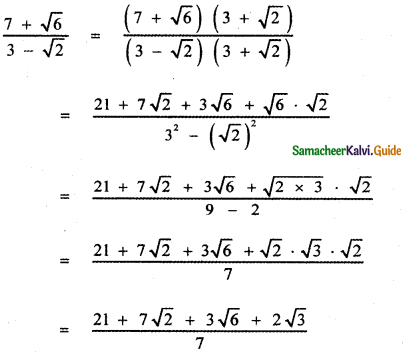Question 7.
Simplify: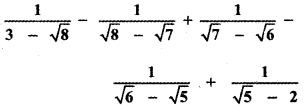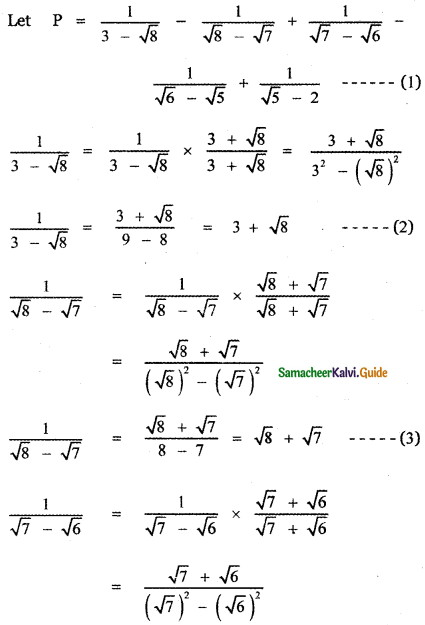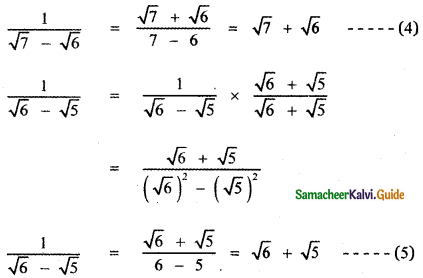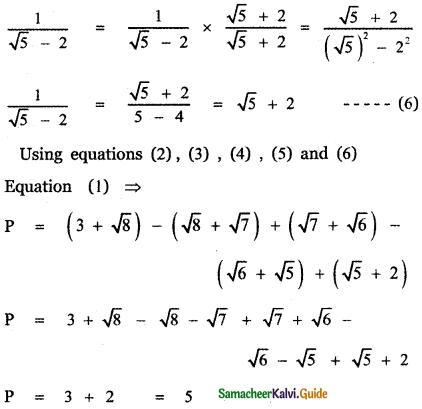Question 8.
If x = √2 + √3 find $$\frac{x^{2}+1}{x^{2}-2}$$